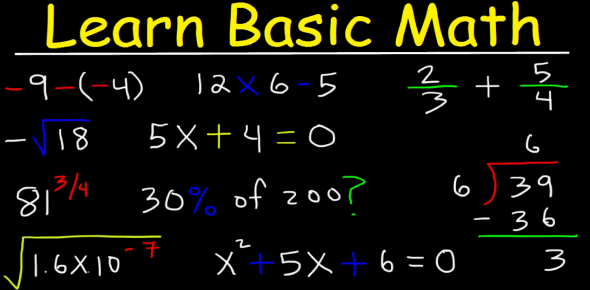# Can You Pass This Basic Math And Arithmetic Quiz?

Approved & Edited by ProProfs Editorial Team
At ProProfs Quizzes, our dedicated in-house team of experts takes pride in their work. With a sharp eye for detail, they meticulously review each quiz. This ensures that every quiz, taken by over 100 million users, meets our standards of accuracy, clarity, and engagement.
| Written by Kevinegidi
K
Kevinegidi
Community Contributor
Quizzes Created: 1 | Total Attempts: 511
Questions: 10 | Attempts: 511SettingsThis math and arithmetic quiz consists of different questions for beginners as well as experts, who love solving mathematical word problems, equations or calculations. Read the questions carefully and take your time. So, let's try out the quiz. All the best!

• 1.

### What basic arithmetic function is seen as successive addition.

• A.

Arithmetic

• B.

Mulitplication

• C.

Division

• D.

Subtraction

• E.

B. Mulitplication
Explanation
Multiplication is the basic arithmetic function that is seen as successive addition. In multiplication, the process involves adding a number to itself a certain number of times. For example, 3 multiplied by 4 is equivalent to adding 3 four times, which results in a total of 12. Therefore, multiplication can be understood as a repeated addition of the same number.

Rate this question:

• 2.

• A.

+

• B.

=

• C.

-

• D.

X

• E.

>

A. +
Explanation
The plus sign (+) represents addition. It is commonly used in mathematics to indicate that two or more numbers or quantities should be added together to find the sum.

Rate this question:

• 3.

### What is 7.57 + 1 ?

• A.

8.57

• B.

8.75

• C.

6

• D.

3

• E.

1

A. 8.57
Explanation
The correct answer is 8.57 because when you add 7.57 and 1, you get a sum of 8.57.

Rate this question:

• 4.

### Which of the numbers below represents the following: Six million, four hundred sixty-two thousand, three hundred fifty-one

• A.

6,351,462

• B.

6,462,000

• C.

6,462,351

• D.

600,462,351

C. 6,462,351
Explanation
The given number, "Six million, four hundred sixty-two thousand, three hundred fifty-one," can be represented as 6,462,351. This is because the number starts with 6 million, followed by 462 thousand, and ends with 351.

Rate this question:

• 5.

### Compare the following numbers and use symbols to show the relationship. 4,912,641 4,931,221

• A.

>

• B.

<

• C.

=

• D.

None of the above

B. <
Explanation
The number 4,912,641 is less than the number 4,931,221.

Rate this question:

• 6.

### Can you order the following numbers from GREATEST TO LEAST using the greater than and less than symbols? 45,999 54,923 223,564 1,344,534

• A.

1,344,524>223,564>54,923>45,999

• B.

223,564>54,923>45,999>1,344,524

• C.

45,99>54,923>223,564>1,344,524

• D.

1,344,524<223,564<54,923<45,999

A. 1,344,524>223,564>54,923>45,999
• 7.

### The fraction 3/8 is equivalent(equal) to which of the following fractions?

• A.

6/24

• B.

9/24

• C.

3/24

• D.

8/3

B. 9/24
Explanation
The fraction 3/8 is equivalent to 9/24 because both fractions can be simplified to 3/8 by dividing the numerator and denominator by 3.

Rate this question:

• 8.

### Which of the following decimals is equivalent to the fraction 3/100?

• A.

3.00

• B.

0.30

• C.

0.03

• D.

0.003

C. 0.03
Explanation
The decimal 0.03 is equivalent to the fraction 3/100 because when we divide 3 by 100, we get 0.03. The decimal point separates the whole number part (0) from the decimal part (03), indicating that we have 3 hundredths.

Rate this question:

• 9.

### APPROXIMATELY how many total eggs are in the box if there are: 62 eggs in one basket 51 eggs in another basket 79 eggs in the last basket

• A.

192

• B.

190

• C.

200

• D.

180

B. 190
Explanation
The total number of eggs in the box can be calculated by adding the number of eggs in each basket together. In this case, there are 62 eggs in one basket, 51 eggs in another basket, and 79 eggs in the last basket. Adding these numbers together gives a total of 192 eggs. However, the correct answer is 190. It is possible that there was a mistake made in counting or recording the number of eggs in one of the baskets.

Rate this question:

• 10.

### Which of the following two is GREATER? 4/10 0.49

• A.

0.49

• B.

4/10

• C.

They are equalBack to top